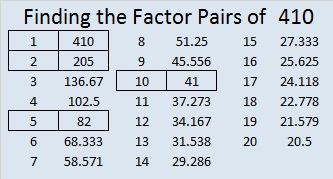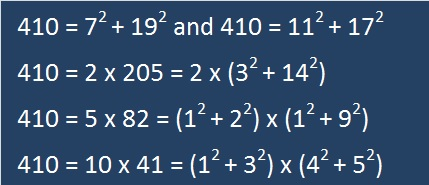# 410 Is The Sum of Two Squares Two Different Ways

410 = 59 + 61 + 67 + 71 + 73 + 79, six consecutive prime numbers.

410 = 199 + 211, two consecutive prime numbers.

• 410 is a composite number.
• Prime factorization: 410 = 2 x 5 x 41
• The exponents in the prime factorization are 1, 1, and 1. Adding one to each and multiplying we get (1 + 1)(1 + 1)(1 + 1) = 2 x 2 x 2 = 8. Therefore 410 has exactly 8 factors.
• Factors of 410: 1, 2, 5, 10, 41, 82, 205, 410
• Factor pairs: 410 = 1 x 410, 2 x 205, 5 x 82, or 10 x 41
• 410 has no square factors that allow its square root to be simplified. √410 ≈ 20.2485Those factors hardly seem like anything to write home about, but wait….How do I know all this? I applied something I read on Dr. David Mitchell’s blog, Lattice Labyrinths. He recently wrote a fascinating post about Tessellations and Pythagorean Triples. You have to read the article or at least look at his tessellations!

I didn’t know multiplying tessellating lattice labyrinths had anything to do with Pythagorean triples, but they do! (Actually I didn’t know anything about tessellating lattice labyrinths until I read the post.)

About the fourth or fifth paragraph of Dr. Mitchell’s post, he states something I didn’t know before: If you take one number that is the sum of two squares and find another number that is also the sum of two squares, and then if you multiply those two numbers together, that product will also be the sums of two squares! Since I knew both 10 and 41 could be written as the sum of two squares, I had to see if 410 could be also. I was doubly surprised and certainly not the least bit disappointed. I don’t know if it’s unusual that most of 410’s factors can also be written as the sum of two squares.

Because 410 and so many of its factors have that property, it is the hypotenuse of four non-primitive Pythagorean triples:

• [266-312-410] is [133 – 156 – 205] times 2.
• [246-328-410] is [3-4-5] times 82.
• [168-374-410] is [84-187-205] times 2.
• [90-400-410] is [9-40-41] times 10.

It is also the short leg of four other triples:

• [410-984-1066] is [5-12-13] times 82.
• [410-1656-1706] is [205-828-853] times 2.
• [410-8400-8410] is [41-840-841] times 10.
• [410-42024-42026] is [205-21012-21013] times 2.

## 5 thoughts on “410 Is The Sum of Two Squares Two Different Ways”

1.abyssbrain

Nice post 🙂 I would also want to add that 410 is the sum of six consecutive primes

410 = 59 + 61 + 67 + 71 + 73 + 79

2.Joseph Nebus

I hadn’t known that about the product of numbers that’re the sums of two squares. That’s rather neat.

•ivasallay

I was aware that it happened some of the time, but not that it is ALWAYS true. After all almost anything can happen some of the time.

3.davescarthin

That makes three of us who didn’t know of the “product of sums of pairs of squares” law. Presumably a coincidence that you looked at my sum of squares post while investigating such a fruitful number as 410, the sum of two squares in two ways.There aren’t going to be many smaller numbers with this property; the first few are 25 (Pythag), 50,65, 85,100 (non-primitive Pythag), 125, 130. Somewhere in my notes I think I have the lowest number that is the sum of squares in THREE ways.

While I’d shown in the Lattice Labyrinths post that you can get Pythagorean triples with 410 as the hypotenuse by “squaring” the number pairs (17,11) and (19,7), I had not realised that you could get another two (as you have done) by multiplying (17,11)x(19,7) and (17,11)x(7,19), and in general where different sums of pairs of squares add up to the same total.

As for the four triples you list with 410 as the smallest of the sides, I can get two of them by letting 410 = 2x205x1 and 2x41x5 = 2ab in Euclid’s formula but I don’t know how you found the other two.

Conway and Guy in the Book of Numbers show how a number can only be the sum of two squares if it and all powers of its prime factors are not of the form 4n-1 (=4m+3). A handy test, which 410 of course passes.

•ivasallay

Thank you for the informative and comprehensive comment! Conway and Guy’s test was not known to me, but will be very helpful to me in the future, I’m sure.

Euclid’s formula produces all of the primitive triples and some of the non-primitive ones. However, a = 2kmn, b = k(m^2-n^2), c = k(m^2+n^2) will produce all triples. I’ve checked all the factor combinations for 410, 984, and 8400 and must conclude that k was not equal to one for the triples with 984 or 8400 as the 2nd number.

This site uses Akismet to reduce spam. Learn how your comment data is processed.